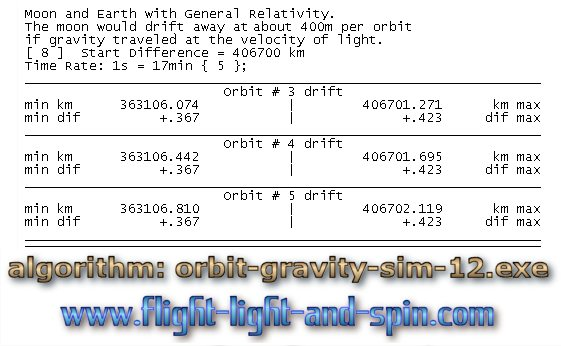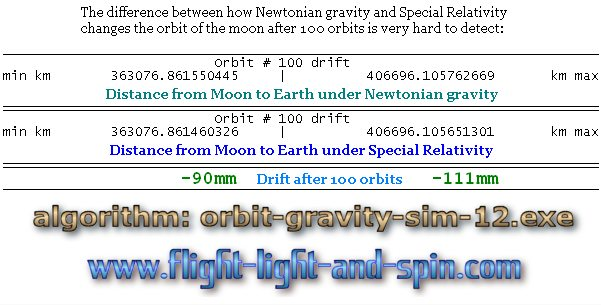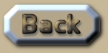Relativity Simulation of the orbit of the Moon

 Relativity and the Moon's orbit Up until now I had been using NASA’s planetary fact-sheet as the most likely source of detailed data. A troubling problem on that web-page is that whilst NASA is making the famous claim of a 36mm recession of the Moon’s orbit from the Earth per year, it also only gives the Moon’s orbit rounded off to the nearest thousand kilometres! A search for more accurate data shows a huge diversity of opinion as to what the Moon’s distance from the Earth actually is. Various websites show discrepancies of many thousands of kilometres for both the nearest and furthest distance. It is quite a raging debate. Some claims are that the eccentricity is changing, the maximum and minimum distances are varying from orbit to orbit; and that this is all a consequence of the other planets and the Sun too. Those details will have to wait for another chapter to be tackled more thoroughly. I do have an algorithm which promises to demonstrate the exact consequences of n-body gravity - but all in good time. So for now the exact details of the Moon’s orbit used by the algorithm orbit-gravity-12.exe (OGS12) will be assumed as being 363 104km to 406 700km. The effect of Relativity on the Moon’s orbit is always going to be proportional. So altering those amounts will not result in different consequences as to how Relativity changes orbits in principle. So let us look at how the Newtonian Scenario  demonstrates a deviation of just a matter of meters even when the moon orbits at a time-rate of 1:100000. This is a nice scenario to observe evolving, because at this scale one can see the diameters of the Moon and the Earth clearly. The wobble that the Moon’s gravity causes on the Earth is also visible on the computer screen here. .
 Also note that Scenario  improves on Scenario  because it has 10 times more sides to the polygon-orbit and thus it demonstrates an error-margin of less than half-a-meter per orbit. Scenario  calculates at the same time-scale as Scenario  which then also adds the velocity of gravity from General Relativity to the orbit of the Moon. Even though it is fairly easy to improve on this error-margin by increasing the number of sides to the orbit-polygon, there is no real need here, because the fluctuation caused by gravity-velocity dwarfs the error-margin by 100 times. The results of Scenario  are superb: .The outwards spiral of 400m per orbit if gravity travels at the velocity of light would be due to the reciprocal delay between the forces of gravity between the two bodies. The Moon would be attracted to a point 16m from the Earth’s center at an earlier point on the orbit-path; whilst the Earth would similarly be pulled by the Moon to a point 1.3km away from the Moon’s center. At this rate, of an outwards spiral of 400m per orbit, the Moon would have separated from the surface of the Earth less than 70 000 years ago - if gravity moved at the velocity of light. So how is the Moon’s orbit influenced by Special Relativity? Scenario  is right on the edge of what is measurable in this algorithm because the velocity of the Moon around the Earth is quite slower (1 km/s) than the velocity of the Earth and Moon together around the Sun (30km/s). And here is where Special Relativity shows a blatant contradiction: If we take the velocity of the Moon to include the velocity that the Earth-Moon system has around the Sun, then the Moon’s velocity is much closer to the velocity of light – and thus it would be more greatly altered by the reduction in velocity from Special Relativity. Here the 100th orbit of the Newtonian Scenario  is compared to the 100th orbit for Special Relativity in Scenario : .This is an extract summary of Chapter XXX of the book: Flight Light and Spin